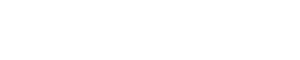Drag

# 机械工程代写｜Mechanical Engineering ME3O04

## 1. First Question of Five (40 Marks)

a) Consider a fully developed laminar, pressure driven, flow between infinite parallel plates with
upper plate moving with constant speed U, as shown in Fig 1. Velocity distribution in such flow
is provided by equation (1) below, where, dp/dx is pressure gradient and  is fluid dynamic
viscosity.

i. Discuss changes in the velocity profile as pressure gradient, dp/dx, is (1) negative, (2)
positive, or (3) zero. Sketch the velocity profile for each case.

ii. Determine expressions for the shear stress, , for three cases mentioned in (i) above.
Indicate location of maximum shear stress for each case. Can  be zero in any of these
cases? Discuss. (12 Marks)b) Discuss types of motion of a fluid particle. Indicate which of these types of motion can occur
simultaneously. Can we determine these types of motion using integral or differential analysis? (8
Marks)

c) What is the difference between an inviscid flow and a viscid flow? Under what conditions can
you deal with a flow as inviscid or viscid? Provide an example for each case. (6 Marks)

d) Discuss restrictions of the application of Bernoulli’s equation. Can you apply Bernoulli’s
equation within a boundary layer? Discuss your answer. (8 Marks).

e) Can a gas (e.g. air) be dealt with as an incompressible fluid? If yes, under what conditions? (6
Marks)

## 2. Second Question of Five (20 Marks)

Water flows at low speed, V1 = 7 m/s, through a circular horizontal tube with inside diameter of
60 mm. A smoothly contoured body of 40 mm diameter is held in the end of the tube where the
water discharges to atmosphere, as shown in Fig 2. Assume flow to be inviscid and assume
uniform velocity profiles at each section. Use water density = 1000 kg/m3. Determine:

i) Exit flow velocity, V2.

ii) Pressure measured by the gage at section (1), P1.

iii) Force required to hold the body, F.

iv) If V1 is to be kept constant at 7 m/s, without calculations, discuss possible changes in P1,
F, and V2 if flow is viscid.

## 3. Third Question of Five (20 Marks)

A large tank of liquid under pressure is drained through a smoothly contoured nozzle of area A.
The mass flow rate, m , is thought to depend on nozzle area, A, liquid density, , difference in
height between the liquid surface and nozzle, h, tank gage pressure, P, and gravitational
acceleration, g.

i) How many independent  parameters can be formed for this problem?

ii) Using , A, and g, as repeating variables, find those  parameters.

iii) It is required to design an experiment using a scaled-down tank, i.e., a model, based on
your answers, what are the conditions that you have to meet in order to establish dynamic
similarity in your experiment.